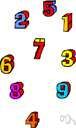# arithmetic progression

Also found in: Thesaurus, Medical, Legal, Financial, Acronyms, Encyclopedia, Wikipedia.
Related to arithmetic progression: geometric progression, harmonic progression

## arithmetic progression

n.
A sequence, such as the positive odd integers 1, 3, 5, 7, ... , in which each term after the first is formed by adding a constant to the preceding term.

## arithmetic progression

n
(Mathematics) a sequence of numbers or quantities, each term of which differs from the succeeding term by a constant amount, such as 3,6,9,12. Compare geometric progression

## arithmet′ic progres′sion

n.
a sequence in which each term is obtained by the addition of a constant number to the preceding term, as 1, 4, 7, 10, and 6, 1, −4, −9. Also called ar′ithmet′ic se′ries.

## ar·ith·met·ic progression

(ăr′ĭth-mĕt′ĭk)
A sequence of numbers such as 1, 3, 5, 7, 9 ..., in which each term after the first is formed by adding a constant to the preceding number (in this case, 2). Compare geometric progression.

## arithmetic progression

- A sequence in which each term is obtained by the addition of a constant number to the preceding term, as 1, 4, 7, 10, 13.
ThesaurusAntonymsRelated WordsSynonymsLegend:
 Noun 1arithmetic progression - (mathematics) a progression in which a constant is added to each term in order to obtain the next term; "1-4-7-10-13- is the start of an arithmetic progression"math, mathematics, maths - a science (or group of related sciences) dealing with the logic of quantity and shape and arrangementpatterned advance, progression - a series with a definite pattern of advance
References in periodicals archive ?
In this paper, we present the theory of completely regular codes in the Hamming graph enjoying the property that the eigenvalues of the code are in arithmetic progression. We call these codes arithmetic completely regular codes and we classify them under some additional conditions.
The best values of the calculated arithmetic progression were obtained by students in the fifth grade, according to their physical skills and they exceed the minimum scale established by SNE with a number of 2.23 repetitions; the calculated arithmetic progression for students in the sixth grade exceed the minimum scale with 2.09 repetitions, and that for students in the seventh grade exceed it with 1.72 repetitions.
It is well known that the sequence a in G is an arithmetic progression of order h if and only if there exists a polynomial p(n) in n, with coefficients in G and of degree less than or equal to h, such that p(n) = [a.sub.n], for every n = 0, 1, 2 ...; that is, there are [[gamma].sub.h], [[gamma].sub.h-1], ..., [[gamma].sub.1], [[gamma].sub.0] [member of] G, which depend only on a, such that, for every n = 0, 1, 2, ...,
we can distinguish an arithmetic progression 1/2, 1/3, 1/6 that consists of three terms.
What could be a more natural arithmetic progression than another in 2009?
Arrhenius said this more than one hundred years ago, "If the quantity of carbonic acid increases in geometric progression, the augmentation of the temperature will increase in nearly arithmetic progression."
The joint with the open root crack was analyzed, the crack lengths being considered in arithmetic progression, with the ratio 0.5 mm.
can be extended analytically to a meromorphic function with poles at arithmetic progression on a negative real semi-axis.
Points P and Q, moving along at different rates in Figure 1, PB decreasing in geometric progression while CQ increases in arithmetic progression, would necessarily fall in the jurisdiction of the mathematics of change, namely calculus.
"124," however, contained "4," a number associated with magic in both West African and Western cultures; and instead of a simple arithmetic progression, each successive number in "124 "doubles the preceding number, creating an open-ended geometric progression (Jeanette K.
Thus, one might reasonably say that the means of subsistence increase by arithmetic progression. Let us now consider the effects of these two types of progression.

Site: Follow: Share:
Open / Close